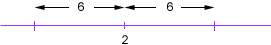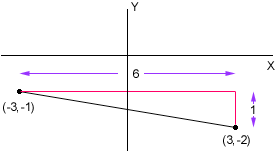hi, my name is Becky, my question is: What are all the real values of x that are solutions for the inequality [x-2] < 6? ( it's less than or equal to) What is the distance between the points with (x,y) coordinates (3,-2) and (-3,-1)? Thanks, Hi Becky, I am not sure how you are using the square brackets in your first problem. If they are just acting as brackets then the solution would be obtained by adding 2 to each side of the inequality. [x-2] <= 6 x - 2 + 2 <= 6 x <= 6 So the answer is all numbers x which are less than or equal to 6. I think however that you might be using square brackets to designate absolute value, that is [x-2] = |x-2|. If this is what you mean then my solution would be to interpret |x-2| as the distance between x and 2 and think of the question geometrically. If |x-2| is less than or equal to 6 then x can't be more than 6 units to the right of 2 or 6 units to the left of 2.From the diagram it is clear that x can be any number between -4 and 8, that is x is any number that satisfies -4<=x and x<=8. For your second problem I would again draw a diagram and this time use the theorem of PythagorasThe triangle in the diagram is a right triangle so the length of the hypotenuse (distance between the points) is the square root of 62 + 12 There is an algebraic formula for the distance between points in the plane but it is just an algebraic way to express the theorem of Pythagoras. Cheers, Penny Go to Math Central